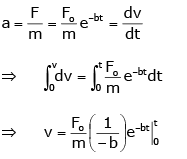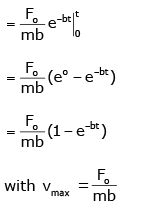# Test: Kinematics (Previous Year Questions Level 1)

## 28 Questions MCQ Test Physics Class 11 | Test: Kinematics (Previous Year Questions Level 1)

Description
This mock test of Test: Kinematics (Previous Year Questions Level 1) for Class 11 helps you for every Class 11 entrance exam. This contains 28 Multiple Choice Questions for Class 11 Test: Kinematics (Previous Year Questions Level 1) (mcq) to study with solutions a complete question bank. The solved questions answers in this Test: Kinematics (Previous Year Questions Level 1) quiz give you a good mix of easy questions and tough questions. Class 11 students definitely take this Test: Kinematics (Previous Year Questions Level 1) exercise for a better result in the exam. You can find other Test: Kinematics (Previous Year Questions Level 1) extra questions, long questions & short questions for Class 11 on EduRev as well by searching above.
QUESTION: 1

### If a body losses half of its velocity on penetrating 3 cm in a wooden block, then how much will it penetrate more before coming to rest ?  [AIEEE 2002]

Solution:

Let initial velocity of body at point A is v, AB is 3 cm.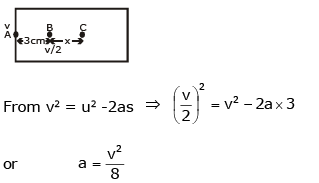Let on penetrating 3 cm in a wooden block, the body moves x distance from B to C.
So, for B to C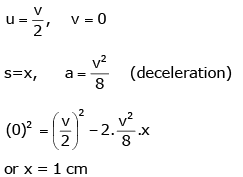NOTE :
Here, it is assumed that retardation is uniform.

QUESTION: 2

### From a building two balls A and B are thrown such that A is thrown upwards and B downwards (both vertically with same velocity). If vA and vB are their respective velocities on reaching the ground,then    [AIEEE 2002]

Solution:

From conservation of energy, potential energy at height h = KE at ground

Therefore, at height h, PE of ball A PE = mAgh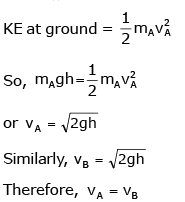NOTE : In question it is not mentioned that magnitude of thrown velocity of both balls are some which is assumed in solution.

QUESTION: 3

### A ball whose kinetic energy is E, is projected at an angle of 45° to the horizontal. The kinetic energy of the ball at the highest point of its flight will be          [AIEEE 2002]

Solution:

At the highest point of its flight, vertical component of velocity is zero and only horizontal component is left which is ux = u cosθ

Given θ = 450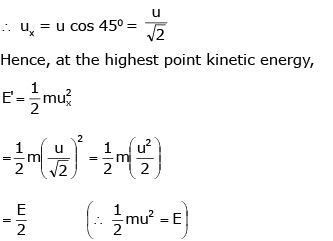QUESTION: 4

Speeds of two identical cars u and 4u at a specific instant. The ratio of the respective distances at which the two cars are stopped from that instant by same braking force :

[AIEEE 2002]

Solution:

In this question the cars are identical means coefficient of friction between the tyre and the ground is same for both the cars, as a result retardation is same for both the cars equal to mg.
Let first car travel distance s1, before stopping while second car travel distance s2, then from v2 = u2 - 2as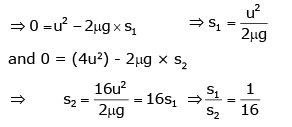QUESTION: 5

The coordinates of a moving particle at any time t are given by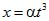and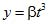.The speed of the particle at time t is given by

[AIEEE 2003]

Solution: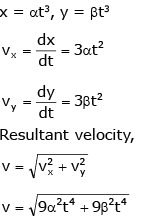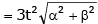QUESTION: 6

A boy playing on the roof of a 10 m high building throws a ball with a speed of 10 m/s at an angle 30° with the horizontal. How far from the throwing point will the ball be at the height of 10 m from the ground ? [g = 10 m/s2, sin 30° = 1/2, cos 30° =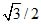]

[AIEEE 2003]

Solution:

As the angle is 30,
cos 30 = √3/2,
Side length = 10,
√3/2 *10 = 8.66

QUESTION: 7

A car moving with a speed of 50 km/h, can be stopped by brakes after at least 6m. If the same car is moving at a speed of 100 km/h, the minimum stopping distance is

[AIEEE 2003]

Solution:

We know that with retardation of a car with initial speed of 50kmph stops after 6m.
We know that v2 = 2as  (third equation of motion)
Thus we get that for some constant retardation, stopping distance is proportional to square of the initial speed
Hence when the initial speed is 100kmph
We get s = 6 x (100/50)2
Thus we get s = 24m

QUESTION: 8

A ball is thrown from a point with a speed v0 at an angle of projection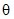. From the same point and at the same instant, a person starts running with a constant speed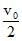to catch the ball. Will the person be able to catch the ball? If yes, what should be the angle of projection ?

[AIEEE 2004]

Solution:

For person to catch the ball they should travel same distance along horizontal axis in time T.

Distance covered by person, R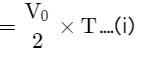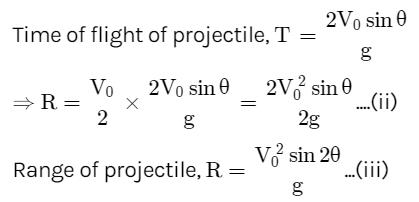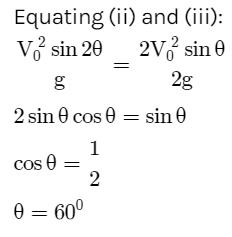QUESTION: 9

A particle is acted upon by a force of constant magnitude which is always perpendicular to the velocity of the particle. The motion of the particle takes place in a plane, it follows that

[AIEEE 2004]

Solution:

When a force of constant magnitude acts on velocity of particle perpendicularly, then there is no change in the kinetic energy of particle. Hence, kinetic energy remains constant.

QUESTION: 10

An automobile travelling with a speed of 60 km/h, can brake to stop within a distance of 20 m. If the car is going twice as fast, i.e. 120 km/h, the stopping distance will be

[AIEEE 2004]

Solution:

We know that with retardation of a car with initial speed of 60kmph stops after 20m.
We know that v2 = 2as  (third equation of motion)
Thus we get that for some constant retardation, stopping distance is proportional to square of the initial speed
Hence when the initial speed is 120kmph
We get s = 20 x (120/60)2
Thus we get s = 80m

QUESTION: 11

A ball is released from the top of a tower of height h metre. It takes T second to reach the ground. What is the position of the ball in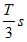?

[AIEEE 2004]

Solution:

Second law of motion gives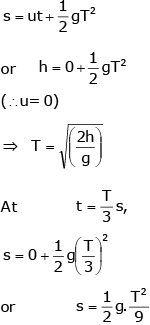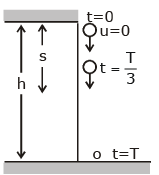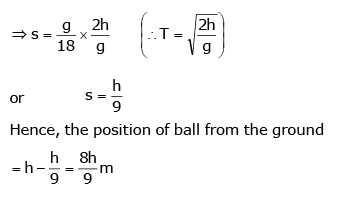QUESTION: 12

A parachutist after bailing out falls 50 m without friction. When parachute opens, it decelerates at 2 ms-2. He reaches the ground with a speed of 3 ms-1. At what height, did he bail out ?

[AIEEE 2005]

Solution:

Parachute bails out at height H from ground. Velocity at A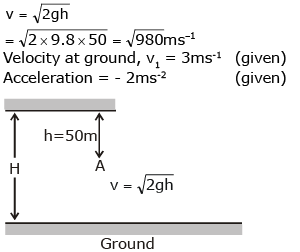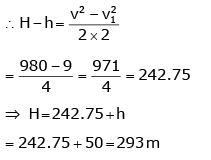QUESTION: 13

A particle is moving eastwards with a velocity of 5 ms-1. In 10 s the velocity changes to 5 ms-1 northwards. The average acceleration in this time is

[AIEEE 2005]

Solution: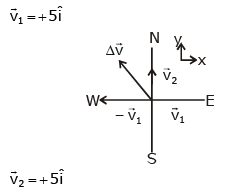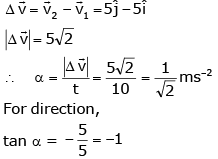Average acceleration is 1/√2 ms-2 towards north - west.

QUESTION: 14

A car, starting from rest, accelerates at the rate f through a distance s, then continues at constant speed for time t and then decelerates at the rate f/2 to come to rest. If the total distance travelled is 15 s, then

[AIEEE 2005]

Solution:

The velocity time graph for the given situation can be drawn as below. Magnitudes of slope of OA = f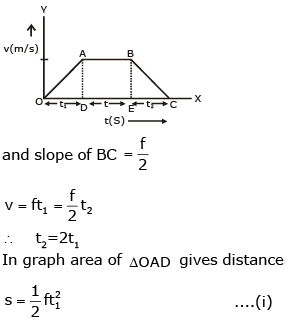Area of rectangle ABED gives distance travelled in time t.

s2 = (ft1)t Distance travelled in time t2,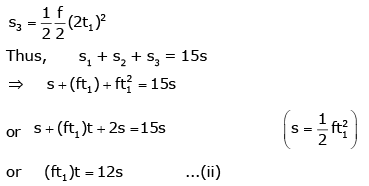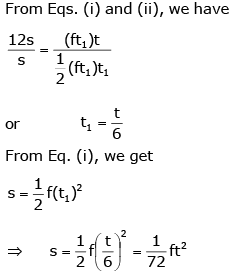QUESTION: 15

The relation between time t and distance x is t = ax2 + bx, where a and b are constants. The acceleration is

[AIEEE 2005]

Solution:

Given, t = ax2+bx

Differentiating w.r.t  t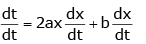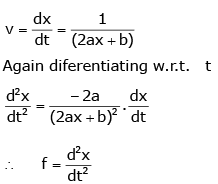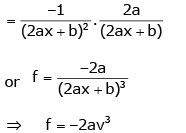QUESTION: 16

A projectile can have the same range R for two angles of projection. If t1 and t2be the times fo flights in the two cases, then the product of the two times of flights is proportional to

[AIEEE 2005]

Solution:

A Projectile can have same range if angle of projection are complementary ie, θ and (900-θ) Thus, in both cases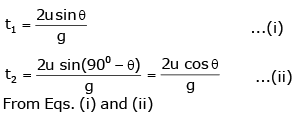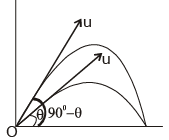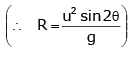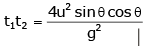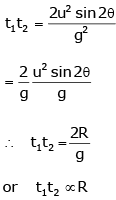QUESTION: 17

A particle located at x = 0 at time t = 0, starts moving along the positive x-direction with a velocity v that varies as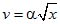. The displacement of the particle varies with time as

[AIEEE 2006]

Solution: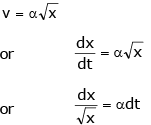Perform integration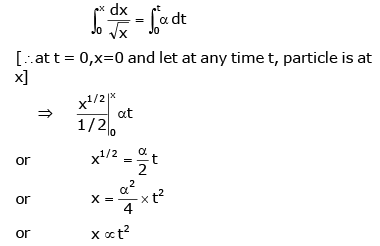QUESTION: 18

A particle is projected at 60° to the horizontal with a kinectic energy K. The kinetic energy at the highest point is

[AIEEE 2007]

Solution:

Kinetic energy at highest point,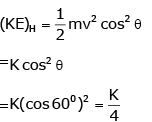QUESTION: 19

The velocity of a particle is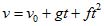. If its position is x = 0 at t = 0, then its displacement after unit time (t = 1) is

[AIEEE 2007]

Solution:

v=v0​+gt+ft2
0x​dx=∫01​(v0​+gt+ft2)dt
⇒x=v0​+g/2​+f​/3.

QUESTION: 20

A body is at rest at x = 0. At t = 0, it starts moving in the positive x-direction with a constant accleration. At the same instant another body passes through x = 0 moving in the positive x- direction with a constant speed. The position of the first body is given by x1 (t) after time t and that of the second body by x2(t) after the same time interval.Which of the following graphs correctly describes (x1 – x2) as a function of time?

[AIEEE 2008]

Solution: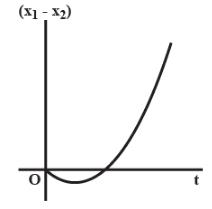x1​(t)=1​/2at2
x2​(t)=vt
⇒(x1+x2) =1/2​at2−vt
Plot (x1​−x2) vs t.

QUESTION: 21

Consider a rubber ball freely falling from a height h = 4.9 m onto a horizontal elastic plate. Assume that the duration of collision is negligible and the collision with the plate is totally elastic. Then the velocity as a function of time the height as function of time will be [AIEEE 2009]

Solution:

v = u + gt. As the ball is dropped, v = gt when coming down. v increases makes collision, the value of v becomes +ve, decreases comes to zero and increases. The change from +v to –v is almost instantaneous. Using –ve signs when coming down, (c) is correct.
Further h = (1/2)gt2  is a parabola. Therefore (c).

QUESTION: 22

A particle has initial velocity (2i+3j) and acceleration (0.31i +0.2j). The magnitude of velocity after 10 s will be

Solution:

From the equation of motion, we knows,
v=u+ at
v= (2i+3j)
a=(0.3i +0.2j)
v=(2i+3j) +(0.3i +0.2j) x 10
v=5i+5j
v=5√2

QUESTION: 23

A small particle of mass m is projected at an angle θ with the x-axis with an intial velocity v0 in the x-y plane as shown in the figure. At a time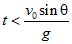, the angular momentum of the particle is

[AIEEE 2010]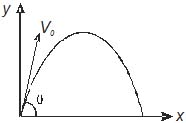Solution: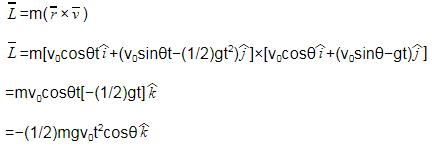QUESTION: 24

A particle is moving with velocity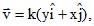where k is a constant. The general equation for its path is

[AIEEE 2010]

Solution:

As,
v= k(yi + xi)
vₓ= Ky so dₓ/dt = Ky
vy= Kₓ so dy/dt = Kₓ
dividing both equations
dy/dₓ= dy/dt divide dx/dt = Kx/Ky =x/y
you will get
ydy= xdx
integrating the equation
y²/2 = x²/2 + c
y2=x2+constant

QUESTION: 25

A water fountain on the ground sprinkles water all around it. If the speed of water coming out of the fountain is v, the total area around the fountain that gets wet is

[AIEEE 2011]

Solution:

Maximum range of water coming out of the fountain

Rm = v2/g

Total area around fountain,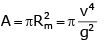QUESTION: 26

An object, moving with a speed of 6.25 m/s, is decelerated at a rate given by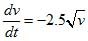where v is the instantaneous speed. The time taken by the object, to come to rest, would be

[AIEEE 2011]

Solution: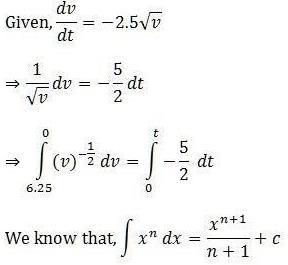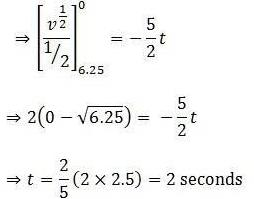QUESTION: 27

A boy can throw a stone up to a maximum height of 10 m. The maximum horizontal distance that the boy can throw the same stone up to will be

[AIEEE 2012]

Solution:

Maximum speed with which the boy can throw stone is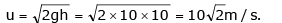Range, is maximum when projectile is thrown at an angle of 450 thus,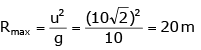QUESTION: 28

A particle of mass m is at rest at the origin at time t = 0. It is subjected to a force F(t) = F0e-bt in the x direction. It speed v(t) is depicted by which of the following curves?

[AIEEE 2012]

Solution:

As the force is exponentially decreasing, so it's acceleration, i.e., rate of increase of velocity will decrease with time. Thus, the graph of velocity will be an increasing curve with decreasing slope with time.